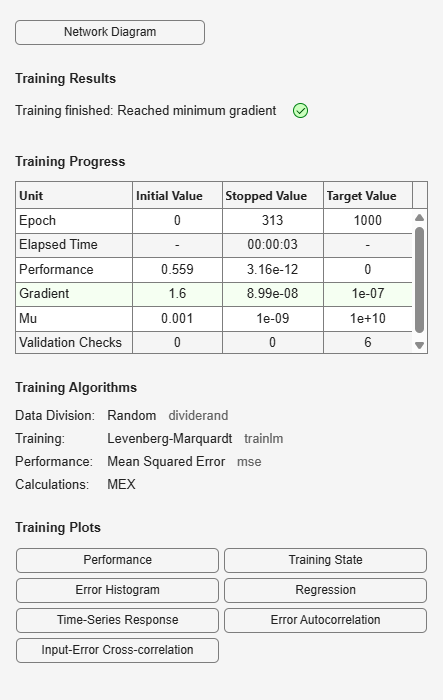# noloop

Remove neural network open- and closed-loop feedback

## Syntax

```net = noloop(net) ```

## Description

`net = noloop(net)` takes a neural network and returns the network with open- and closed-loop feedback removed.

For outputs `i`, where `net.outputs{i}.feedbackMode` is `'open'`, the feedback mode is set to `'none'`, `outputs{i}.feedbackInput` is set to the empty matrix, and the associated network input is deleted.

For outputs `i`, where `net.outputs{i}.feedbackMode` is `'closed'`, the feedback mode is set to `'none'`.

## Examples

collapse all

This example shows how to create and train a NARX network. The NARX network has a standard input and an open-loop feedback output to an associated feedback input.

```[X,T] = simplenarx_dataset; net = narxnet(1:2,1:2,20); [Xs,Xi,Ai,Ts] = preparets(net,X,{},T); net = train(net,Xs,Ts,Xi,Ai);````view(net)``Y = net(Xs,Xi,Ai);`

Now the network is converted to no loop form. The output and second input are no longer associated.

```net = noloop(net); view(net)``````[Xs,Xi,Ai] = preparets(net,X,T); Y = net(Xs,Xi,Ai);```

## Version History

Introduced in R2010b